• 검색 결과가 없습니다.

# Ballistic Carrier Transport Through Graphene : Out-of-Plane and In-Plane Aspects

N/A
N/A
Protected

Share "Ballistic Carrier Transport Through Graphene : Out-of-Plane and In-Plane Aspects"

Copied!
231
0
0
더 보기 ( 페이지)

전체 글

Optical excitation of electrons from the Fermi level of a metal or localized states. Optical microscopic images of the Al/Si contact formed on the Si-trench under Al2O3.

## Introduction

Motivation

### Overview

• Ballistic Carrier Transport
• Out-of-plane Ballistic Carrier Transport through Graphene
• In-plane Ballistic Carrier Transport through Graphene

The Fermi level pinning effect at the metal/graphene/semiconductor junction interface is deeply investigated by modulating the dependence of the energy barrier on the work function of the metal, the metal-graphene interaction, and the state of the semiconductor surface. Schematic illustration of out-of-plane ballistic carrier transport through graphene. a) Hot carrier injection into graphene.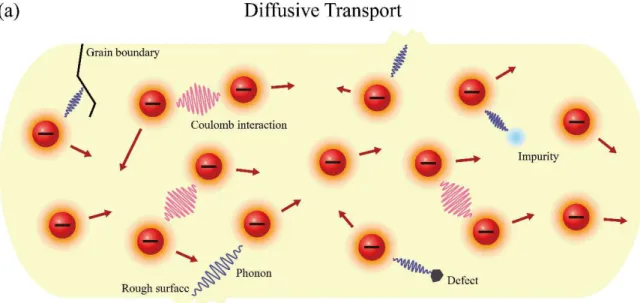Figure 1. Schematic illustration of comparison between the diffusive (a) and the ballistic (b) transport in solid

### Graphene

• Research Trend in Graphene
• Massless Dirac Fermion in Graphene

Due to triangular two equivalent sublattices in hexagonal honeycomb lattice (A and B two carbon atom base Bravais lattice), the electronic states in the graphene are given by the Dirac equation (Equation 2)  with coupling between the momentum and the pseudo- spin [15-23], where σ ˆ is the Pauli matrix consisting of 0 1. The associated Hamiltonian equations are expressed as Equation 4 for the kinetic energy and Equation 5 for the total energy, where U is the potential energy .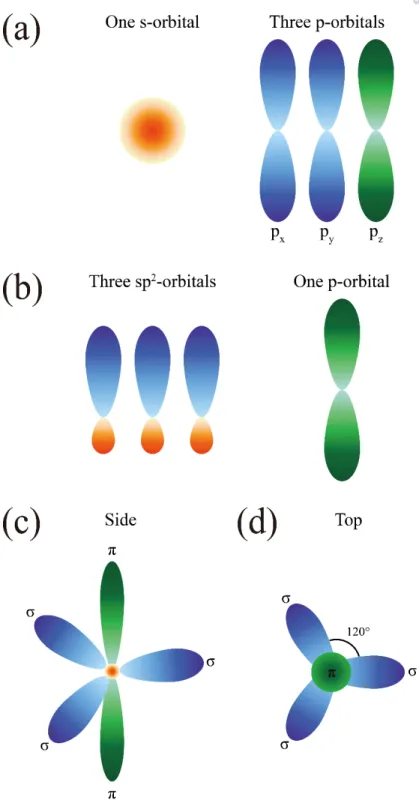Figure 4. Orbital configuration and the bonding of the graphene. (a) Orbitals of a carbon atom

### Metal/Semiconductor Junction

• Energy Barrier Formation
• Fermi-Level Pinning Effect
• Image Force Lowering
• Transport Mechanism
• Parallel Conduction Model

The most commonly used in analysis of the current-voltage characteristic measured on the Schottky contact is the thermionic emission, which is followed by tunneling and recombination. In other words, the Schottky barrier height φB0 at zero bias will be φB + ∆ φ, where ∆φ is the image power lowering energy.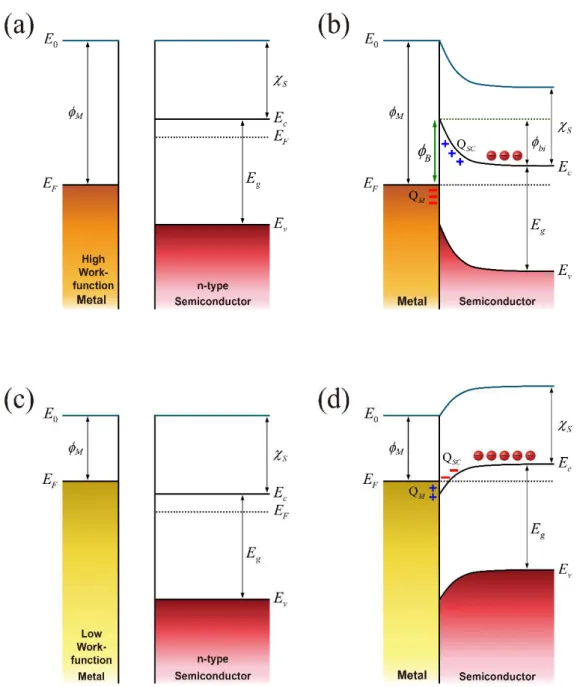Figure 7. Energy band alignments of the junction formed with metal and n-type semiconductor showing how the Schottky (a,b) or Ohmic (c,d) contacts are determined by the Schottky barrier height and how it depends on the high and low

### Internal Photoemission

• Basic Principle
• Experimental Setup

To obtain the quantum efficiency of IPE, the dependence of the measured photocurrent on the photon energy with the light power must be clarified. The injection of an optically excited electron can directly overcome (a) or pass through (b) the interfacial energy barrier. a,b) Optical excitation of an electron from the Fermi level EF of a metal (a) or localized electronic states around the charge neutrality level ECNL of a semiconductor (b) for a metal/semiconductor junction, where Ec is the conduction band edge in the semiconductor and Ev.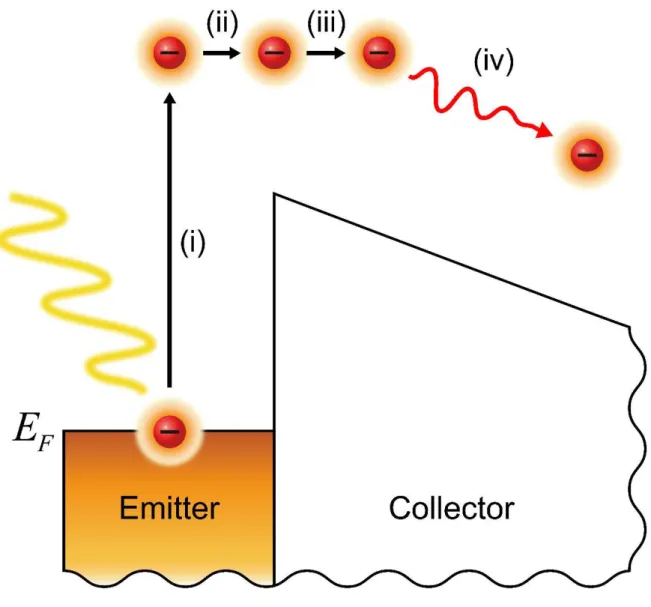Figure 14. IPE process explained by the multi-step model  around the very near interface between emitter and collector, where E F is the Fermi-level

### Graphene Hetero-Interface

• Graphene-Metal Interaction
• Graphene Diffusion Barrier
• Graphene Interlayer

QD is the dipole charge density, QG is the graphene doping charge density, and d is the van der Waals distance between the graphene and the metal. EF is the Fermi level, ∆ EF is the Fermi level shift in the graph, E0 is the vacuum level.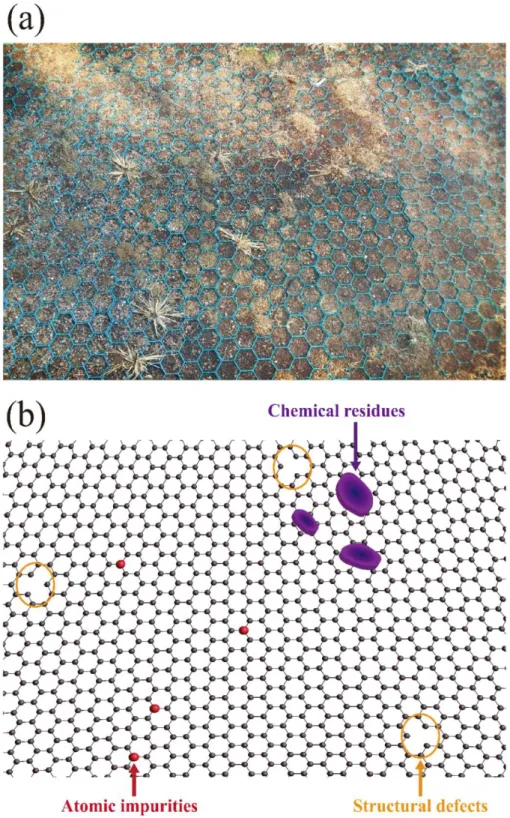Figure 26. (a) Photo taken near the playground showing the hexagonal mesh structure. (b) Graphene lattice structure drawn with chemical residues, atomic impurities, and structural defects, which is inspired by the photo

### Dirac Fermion Quantum Optics in Graphene

• Klein Tunneling in Graphene
• Superlattice Pattern in Graphene

The low Schottky barrier height of the low barrier regions [359–377] is assumed to be the Schottky barrier height extracted from the first IPE threshold (Figure 35a), and the high Schottky barrier height of the predominant high barrier region is assumed to be the Schottky barrier height extracted from the second IPE threshold (Figure 35a). Using the idea of ​​the graphene diffusion barrier (Chapter 1.6.2) and the graphene interlayer (Chapter 1.6.3), the theoretically predicted strong Fermi level pinning effect at the metal/Si interface was experimentally explored (Chapter 2).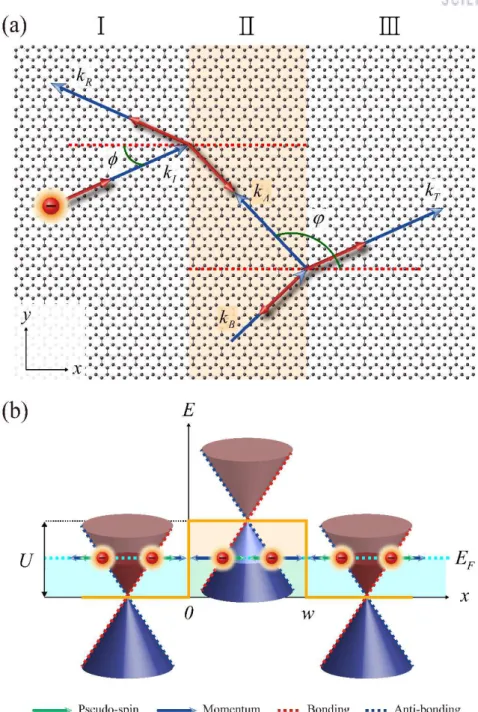Figure 30. (a) Schematic of the electron in the graphene incident on the sharp square potential barrier of height U and width w , where φ is the incident angle, ϕ is the transmitted angle escaping from the potential barrier, k I

## Strong Fermi-Level Pinning Effect at Metal/Graphene/Si Junction

### Sample Fabrication

The graphene on the bottom must be removed by reactive ion etching (RIE) at a power of 10 W for 20 seconds under a pressure of 10 mTorr, maintained by 50 sccm of O2. Then, the graphene/Si sample should also be rinsed with isopropanol and heated again at 200 ◦C for 15 minutes.

Current-Voltage Measurement

Internal Photoemission Measurement

Transmission Electron Microscopy Image

Parallel Conduction Model Calculation

Strong Fermi-Level Pinning Effect

### Fermi-Level Pinning Effect Depending on the Junction Area

The influence of the interface electric dipole layer can be very limited near the interface when the area of ​​the electric dipole layer is small (b,d).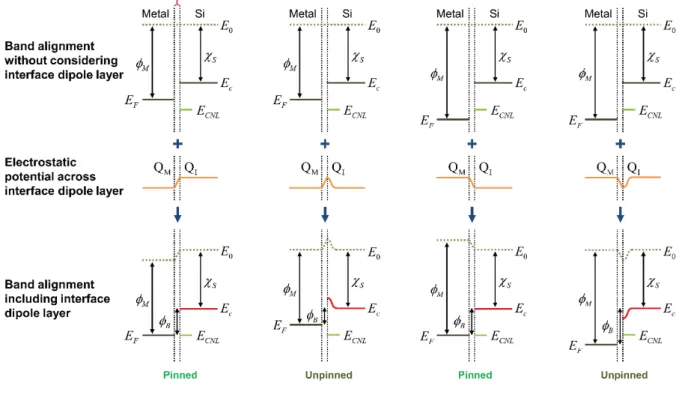Figure 39. (a-d) Band alignment at the very near the metal/Si interface for large (a,c) and small (b,d) junction areas showing the change of electrostatic potential across interface electric dipole layer [15, 177, 189, 190, 207-224]

### Conclusion

As shown in Figure 61 and Figure 62a, the recombination current in the graphene/Si junction seems to survive at the low temperature up to about 100 K. On the other hand, for the Al/graphene/Si junction (Figure 70b and Figure 70d) , the recombination is expected to become significant with the graphene interlayer.

## Negative Fermi-Level Pinning Effect at Metal/Graphene/GaAs Junction

### Sample Fabrication

As depicted in Figure 41, the CVD-grown graphene monolayer purchased from Graphene Supermarket is transferred to the GaAs surface. The exposed graphene from the metal electrode is removed by RIE to isolate any metal/graphene/GaAs junctions. a-c) Raman spectra measured on the bare GaAs substrate (a), graphene on GaAs substrate (b) and graphene with the GaAs background signal subtracted (c.

Current-Voltage Measurement

### Internal Photoemission Measurement

The temperature dependent Schottky barrier height and the ideality factor are shown in Figure 59b for the graphene/Si junction and Figure 59c for the Pt/Si junction. The Al/Si and Al/graphene/Si junctions are formed on the graphene-coated and graphene-coated regions, respectively.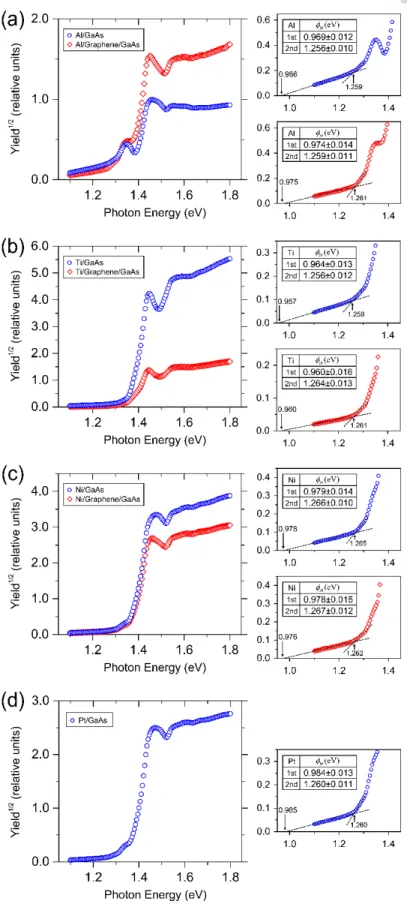Figure 44. (a-d) IPE quantum yield spectra on the metal/GaAs junctions with and without the graphene interlayer for Al (a), Ti (b), Ni (c), and Pt (d) electrodes 

Optical Excitation from Interface-trap states

### Transmission Electron Microscopy Image

High-resolution transmission electron microscopy images taken at several different locations in the Pt/GaAs (Figure 46b and Figure 46c) and Pt/graphene/GaAs (Figure 46e and Figure 46f) junctions also confirm that the thickness of the native oxide layer is spatially inhomogeneous. The thickness of the oxide layer is estimated to be about 2.82 nm for the Pt/GaAs junction and about 2.27 nm for the Pt/graphene/GaAs junction .

### Negative Fermi-Level Pinning Effect

The observed negative effect of Fermi level pinning on I-V measurements is expected to occur here, small spots without native oxide layer. According to the negative Fermi level pinning effect, in the case of the metal/graphene/GaAs junction, the low work function metal electrodes will lead to the formation of relatively higher Schottky barrier heights in the small parts without the native oxide layer than that in the surrounding regions.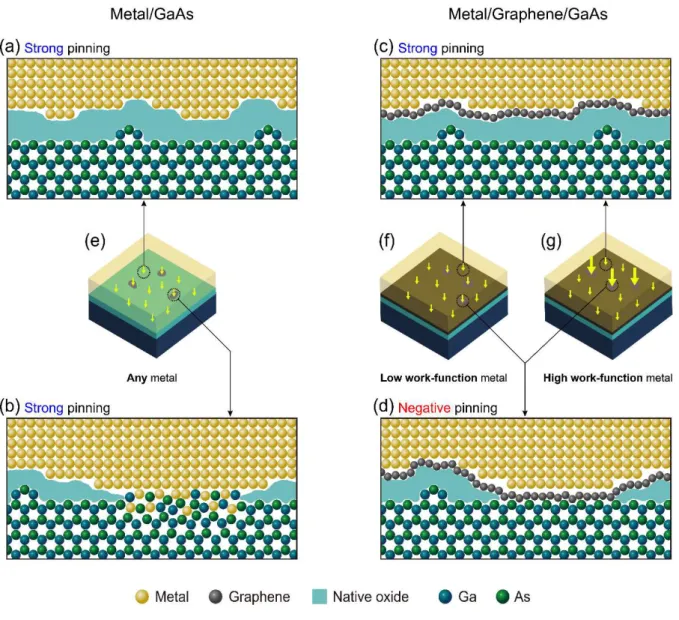Figure 48. (a-d) Atomic arrangements at the metal/GaAs and metal/graphene/GaAs interfaces

### Energy Band Profile across Interface

E x = φ − − χ qU x is the conduction band edge of the GaAs substrate, EF is the Fermi level. Dit is the interstitial trap density on the surface of the semiconductor, and χS is the electron affinity of the semiconductor .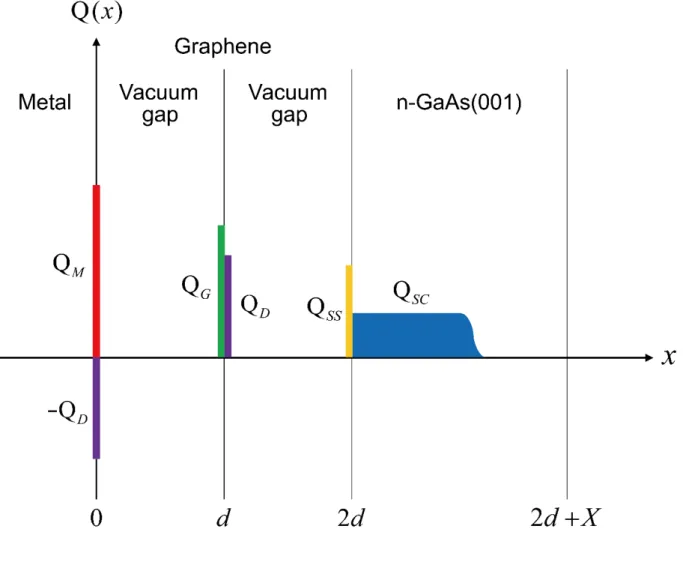Figure 49. Charge distribution across the metal/graphene/GaAs junction , where Q M is the metal surface charge density, Q D is the interaction dipole charge, Q G is the doping charge density of the graphene, Q SS is t

### Electronegativity Difference and Interaction Dipole Charge

It is known that the exchange correlation potential for electrons is attractive to the metal surface, that is, the metal attracts the electrons on the metal surface. Here we can think of the electronegativity difference between the metal and the carbon atom.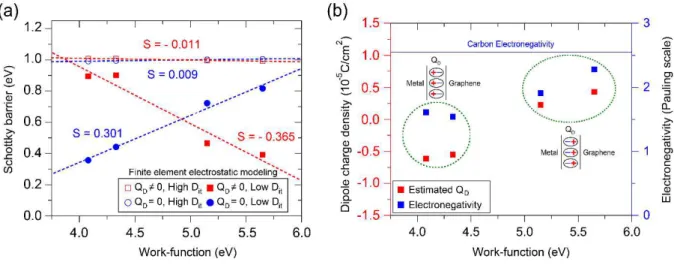Figure 52. (a) Calculated Schottky barrier height φ B of metal/graphene/GaAs junction from the finite element electrostatic modeling as a function of the metal work-function [191-194] for the low interface-trap density D it = 5×10 12

### Parallel Conduction Model Calculation

The blue dashed line shows the estimation of the low-barrier part to match the calculated effective Schottky barrier with the Schottky barrier height sampled from the I-V measurements. The high barrier high for the predominant region is 1.020 eV for (a)-(d), which is assumed to be identical to the Schottky barrier taken from the IPE measurements.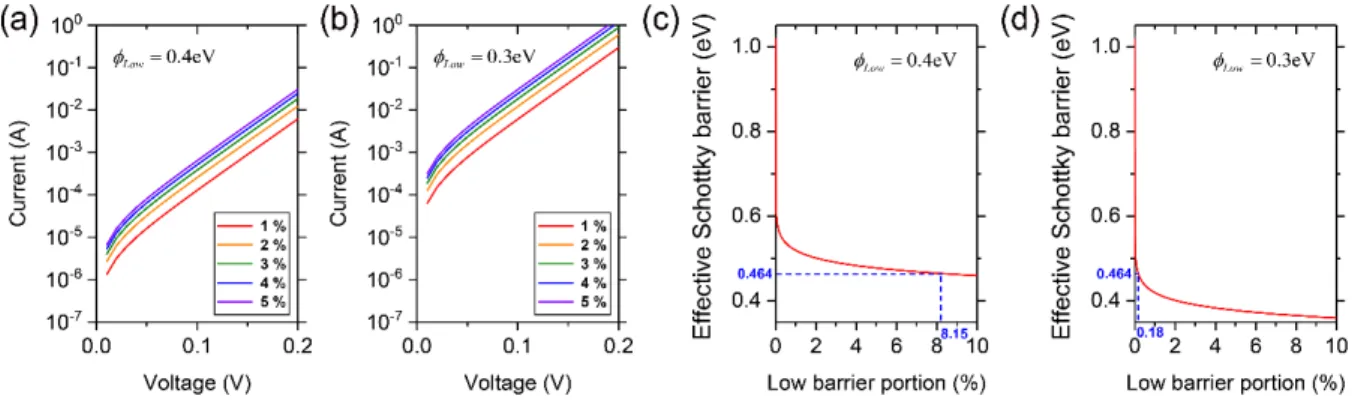Figure 53. Parallel conduction model calculation for the Ni/graphene/GaAs junction. (a,c) I-V curves of the Ni/graphene/GaAs junction for several different low-barrier portion

### Conclusion

As shown in Figure 57j, the drain-to-source channel current in the graphene field-effect transistor fenced by the Si depletion layer (Figure 57d) reveals the low-efficiency switching characteristics, which are likely to be disrupted by the current leakage through the graphene/Si contact (Figure 57e and Figure 57k). Based on the recent studies that claim that the recombination current [354–358] dominates the non-ideal behavior of the forward-biased current in the graphene/Si junction, the measured data are well reproduced with the sum of the thermionic emission current and recombination current by adopting.

## Recombination Enabling Low Temperature Rectification at Graphene/Si Junction

### Sample Fabrication

The graphene field-effect transistor fenced with the Si depletion layer without the SiO2 insulating layer can be fabricated with the next step. The rectangular graphene channel connecting the metal electrodes was formed with the e-beam lithography and the RIE etching. the graphene channel between the metal electrodes is contacted with the Si surface and fenced with not the common SiO2 insulating layer, but the Si depletion layer. a-d) Optical microscope image with the position indicator taken on the graphene on Al2O3. i) Raman spectrum of graphene with the Al2O3 background signal subtracted. j) Raman spectrum of graphene with the Si background signal subtracted. a) Schematic illustration of device structure.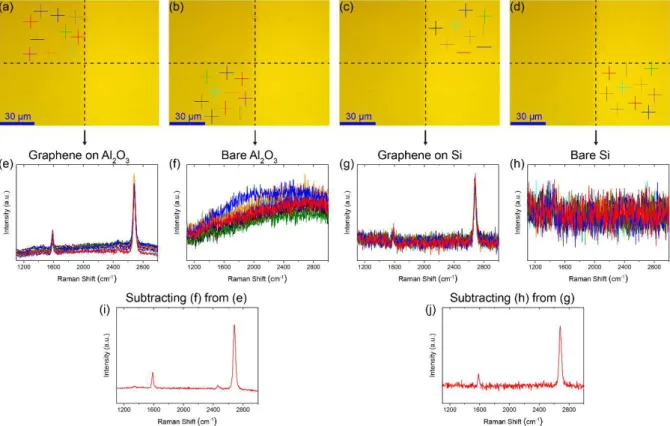Figure 55. (a-d) Optical microscope image with the position indicator taken on the graphene on Al 2 O 3

### Temperature-Dependent Current-Voltage Measurement

Device structure with geometric parameters, where W is the width of the graphene channel, L is the length of the graphene channel, S is the contact length with Si, DL is the length of the graphene contact with the Ti/Au electrode on the left, and DR is the length of the graphene contact with the Ti/Au electrode on the right.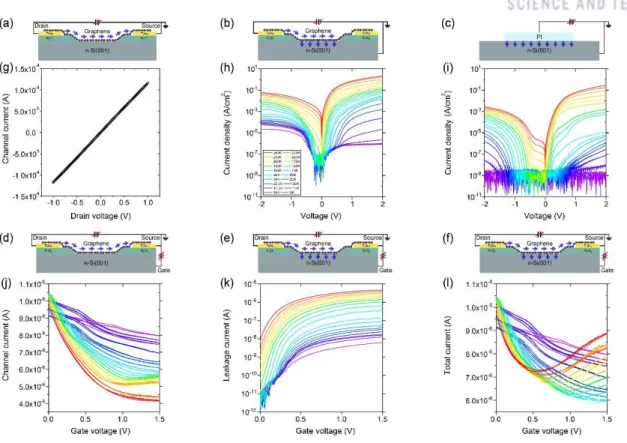Figure 57. (a-f) Device structure and measurement configuration. (g) Graphene channel current versus drain voltage

Improved Rectification Ratio at Low Temperature

### Thermionic Emission Versus Recombination

The cubic temperature T3 is in the coefficient of the equation for the current density of the thermionic emission JTEG [499. Conductivity fluctuations involving localization and confinement can also be observed in graphene with a quantum interference signature [103-122].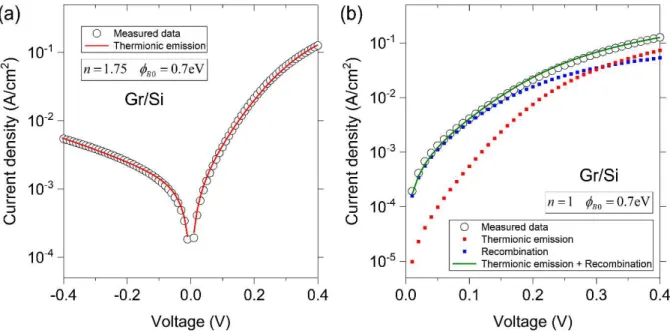Figure 60. (a,b) Current density-voltage curve for the graphene/Si junction. (a) Measured data with the fitting curve to the thermionic emission current density J TEG with n = 1.75 and φ B 0 = 0.7

Recombination Current due to the Hole Carriers Supplied from Grpahene

### Conclusion

Based on the electric dipole layer formed by graphene-metal interaction (Chapter 1.6.1), the unusual negative Fermi level pinning effect at the metal/GaAs interface (Chapter 3) was also observed with the graphene interlayer (Chapter 1.6.3). , which is easily new discovery of unusual nature in device physics. Regarding the in-plane ballistic carrier transport (Chapter 1.2.3), the ballistic carrier transport in the graphene below the superlattice was investigated by introducing the useful concepts in electron quantum optics (Chapter 1.7).

## Enhanced Thermionic Emission and Tunneling at Junction Edges

### Sample Fabrication

After the build-up process, Raman spectroscopy is performed near the Al electrodes in the Si channel. The size of the junction contact is controlled by the lateral width of the Al electrode bonded to the Ti/Au pad on the Al2O3.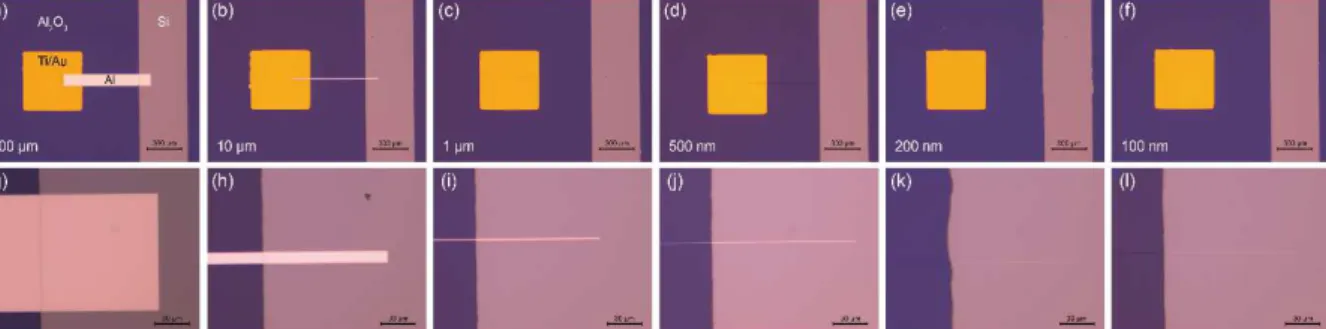Figure 64. (a)-(l) Optical microscope images of the Al/Si junction formed on the Si-trench under the Al 2 O 3

Current-Voltage Measurement

### Energy Band Profile across Interface

The gray rectangles in (g)-(k) represent the areas of the Al electrode. a) – (d) Conduction energy band profiles across the center and edge of the Al/Si junction by varying the lateral width of the Al electrode.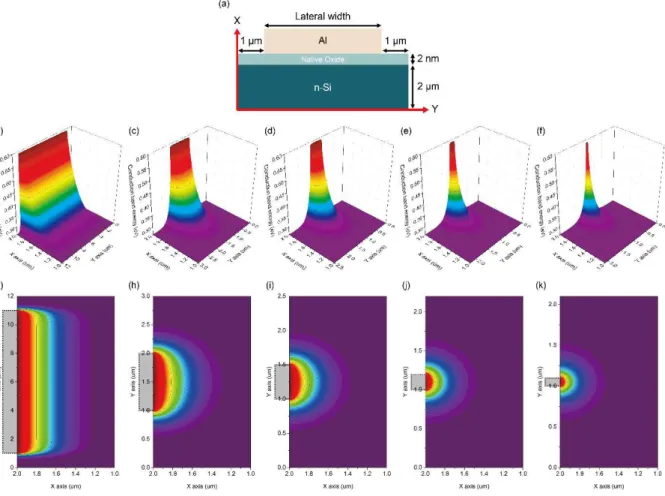Figure 67. (a) Schematic of device structure employed for the modeling. (b)-(k) Conduction energy band distributions at around the Al/Si interface by varying the lateral width of Al electrode

Thermionic Emission Versus Tunneling

Edge Current Enhanced with Downscaling of Lateral Width

### Conclusion

The incident angle is 30° and the size of the superlattice potential is moderate (a), extensive (b), small (c). a,b,c) Scheme of electron tracks entering the graphene superlattice (Chapter 1.7.1 and Chapter .1.7.2). Time-integrated electron wavepacket probability density distributions in a graphene superlattice device with different half-period lengths (150 nm, 50 nm, 30 nm, and 10 nm) by varying the applied voltage (0.1 eV, 0.3 eV, 0.5 eV, and 0 .7 eV) to the superlattice potential barrier.

## Electrically-Controlled Carrier Guiding with Graphene Superlattice

### Device Geometry

136] Huard, B.; Sulpizio, J.; Stander, N.; Todd, K.; Yang, B.; Goldhaber-Gordon, D., Transport measurements across a tunable potential barrier in graphene. 169] Fisichella, G.; Greco, G.; Roccaforte, F.; Giannazzo, F., Current transport in graphene/AlGaN/GaN vertical heterostructures investigated at the nanoscale.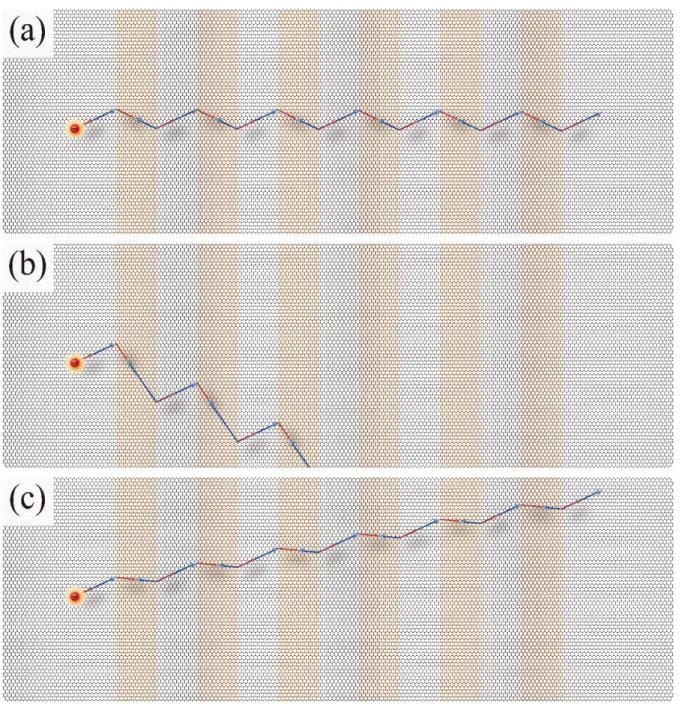Figure 73. (a,b,c) Schematic of trajectories of electron incident to the graphene superlattice (Chapter 1.7.1 and Chapter .1.7.2)

Elctron Trajectory Incident to Graphene Superlattice Potential

### Time-Evolving Electron Probability Distribution in Graphene Superlattice

The Gaussian wave packets Ψ of the massless Dirac fermion in the graphene are given by equation 60, where A is the wave function coefficient, kx is the momentum wave vector component along the x direction, ky is that along the y direction, = 30 nm is the spatial extent along the x, Y = 30 nm is the spatial extent along the y, s is the band index (1 for the conduction band and -1 for the valence band), ui jn, is the first row column component, vi jn, is the column component of the second row, and U is the potential size. Time-evolving probability density distributions of electron wave packets in the graphene superlattice device with half period length of 150 nm by varying the applied voltage (0.1 eV, 0.3 eV, 0.5 eV and 0.7 eV) towards the potential barrier of the superlattice at 100 fs, 150 fs, 200 fs and 250 fs.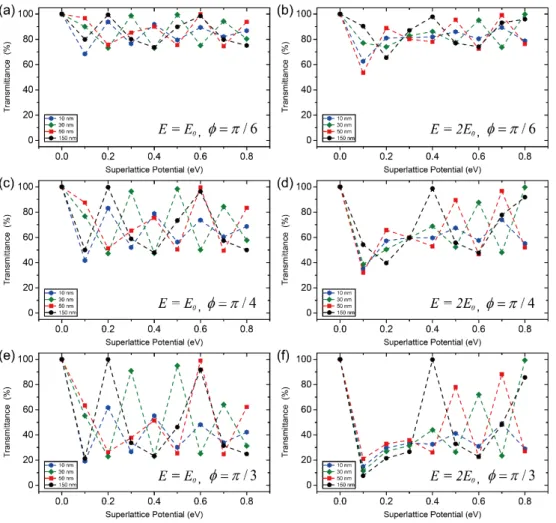Figure 75. (a-f) Transmission coefficient for the electron wave packect incident on the graphene superlattice with the different lengths of half- period (150 nm, 50 nm, 30 nm, and 10 nm) within the same channel length as a function of

Time-Integrated Electron Probability Distribution in Graphene Superlattice

### Superlattice-Periodicity-Dependent On-Off Current Ratio

Drain current ratio (drain current over total current) as a function of the magnitude of the superlattice potential with the different lengths of half-period (150 nm, 50 nm, 30 nm and 10 nm). As shown in Figure 82, the on-off current ratio is obtained as the ratio of the maximum and minimum number of electrons flowing into the drain electrode based on the current ratio in Figure 81.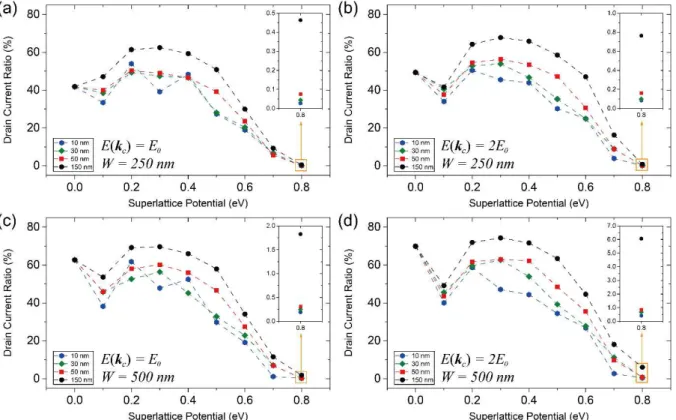Figure 81. Drain current ratio (drain current over total current) as a function of the magnitude of superlattice potential with the different lengths of half- period (150 nm, 50 nm, 30 nm, and 10 nm)

Conclusion

## Graphene Superlattices

As discussed in Chapter 1.7.2, the well-designed superlattice structure formed on the graphene channel functions as an interconnected network for current flow. As shown in Figure 86, by addressing artificially patterned square or triangular superlattices in the graphene channel, the transport path of charge carrier can be modulated depending on its width, interval, shape and periodicity. a,b) Optical images taken on the graphene channel (a) with the superlattice structure (b).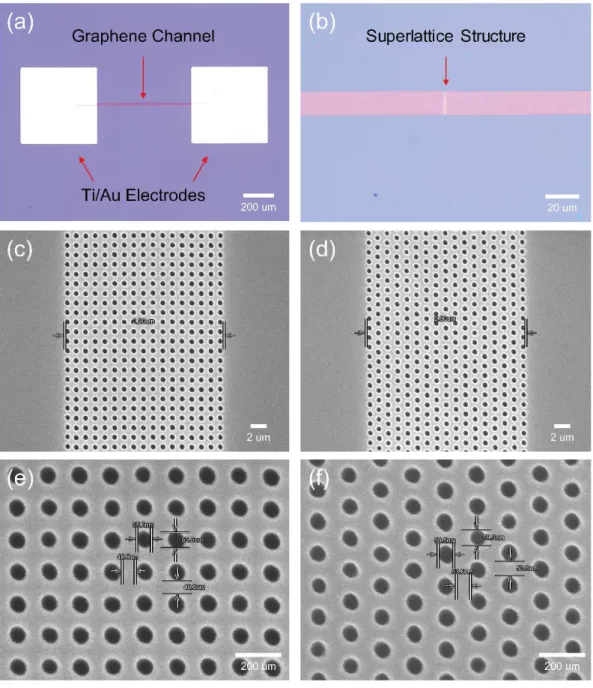Figure 86. (a,b) Optical images taken on the graphene channel (a) with the superlattice structure (b)

Artificial Randomized Defects with Metal Adatom on Graphene

## Graphene Aharonov-Bohm Inteferometer

In this dissertation, two different aspects of ballistic carrier transport (Chapter 1.2) through graphene (Chapter 1.3) are studied (Figure 90). J.; Makarovsky, O., Vertical Field-Effect Transistor Based on Graphene-WS2 Heterostructures for Flexible and Transparent Electronics.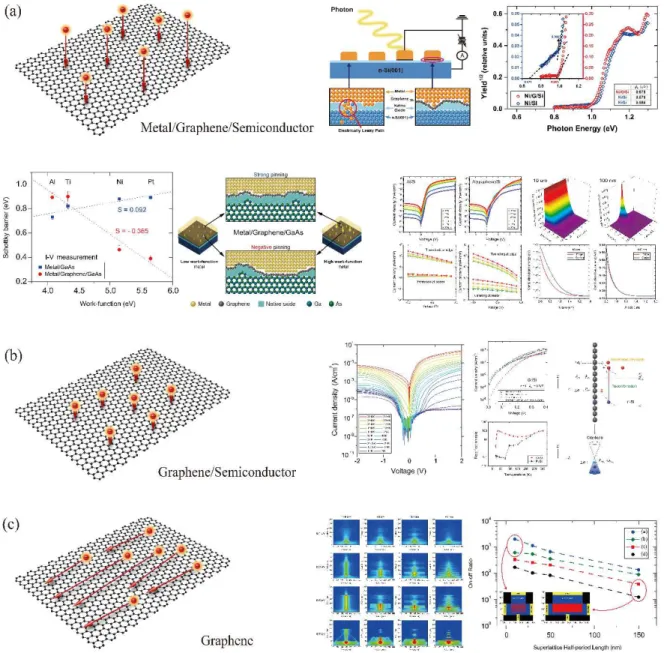Figure 90. Summary and outline of dissertation. (a,b) Out-of-plane ballistic carrier transport through grapehene (a) Ballistic carrier transport across metal/graphene/semiconductor intrerface

수치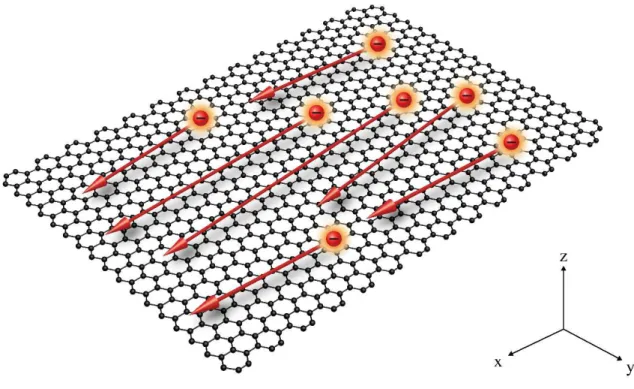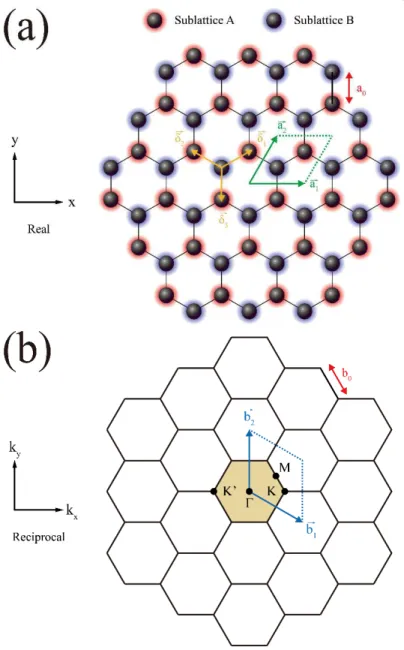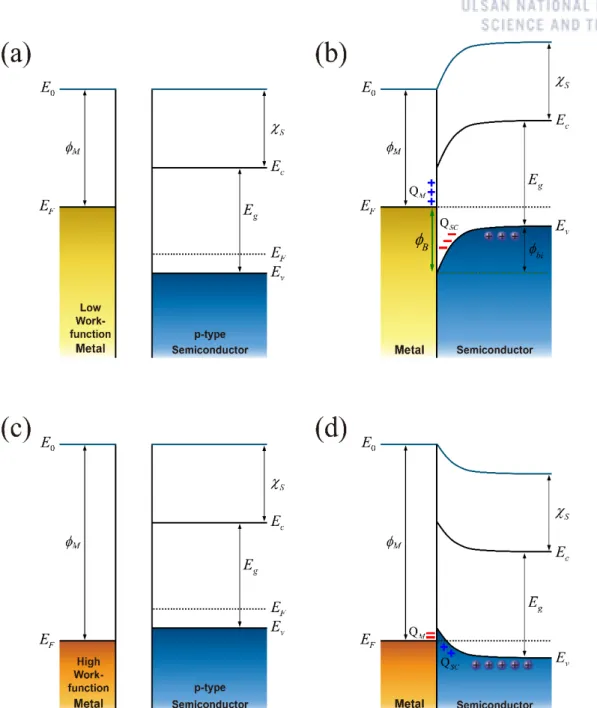+7

참조

관련 문서

The Asia-Montana Energy Summit (AMES) is a strategic initiative led by the Maureen and Mike Mansﬁeld Center to address the complex issues surrounding the future of energy

The kinetic solvent isotope effects determined in water and methanol are consistent with the proposed mechanism of the general base catalyzed and/or S A N/ S N

Figure 5 shows the comparison of J-V characteris- tics between the results from device simulation and SPICE circuit simulation using the Verilog-A as a pa- rameter of

In a laser diode, laser oscillation arises at the wavelength with the maximum gain, which is determined by the band gap energy of the semiconductor since the band gap

① The metal and semiconductor are assumed to be in intimate contact on an atomic scale, with no layers of any type (such as an oxide) between the components. ② There

The obtained results suggest that poly-vinyl caprolactam (PVCap) solution shows elongated growth period than pure water until distinct torque change is observed, which

By applying a sufficiently large positive gate voltage, we have inverted the surface of the semiconductor from a p-type to an n-type semiconductor.... MOS Capacitor :

The activation energy

Contribution to the Global Goals and other government commitments (achieved as at March 2017) * 93 thousand people with sustainable access to clean water and/or sanitation.. 78

언어 선택하기

웹사이트는 선택한 언어로 번역됩니다.

기타 언어